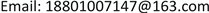MSEManagement Science and Engineering2167-664XScientific Research Publishing10.12677/MSE.2019.81009MSE-29180MSE20190100000_84387547.pdf经济与管理 物流金融风险评估模型研究 Logistics Financial Risk Assessment Model Research 铭铭21世民21北京工商大学计算机与信息工程学院，北京null2901201908016471© Copyright 2014 by authors and Scientific Research Publishing Inc. 2014This work is licensed under the Creative Commons Attribution International License (CC BY). http://creativecommons.org/licenses/by/4.0/Copyright © 2019 by author(s) and Hans Publishers Inc.1. 引言

2. 物流金融信用风险指标体系构建

Logistics financial risk assessment index syste

3PL融资企业基本素质企业规模

Three-level indicators and index descriptio

Indicators with large correlation coefficien

3. 基于支持向量机物流金融信用风险评估模型

3.1. 数学建模

1) 线性分类：

max Q ( α ) = ∑ i = 1 n α i − 1 2 ∑ i = 1 n ∑ j = 1 n α i a j y i y j x i T x j

s.t. ∑ i = 1 n a i y i = 0 ，且 a i ≥ 0 , i = 1 , 2 , ⋯ , n

max Q ( α ) = 1 2 ∑ i = 1 n ∑ j = 1 n α i a j y i y j x i T x j − ∑ i = 1 n α i

s.t. ∑ i = 1 n a i y i = 0 ，且 a i ≥ 0 , i = 1 , 2 , ⋯ , n

s.t. ∑ i = 1 n a i y i = 0 ，且 0 ≤ a i ≤ C , i = 1 , 2 , ⋯ , n

min ω , b , ξ ϕ ( ω , x ) = 1 2 ω T ω + C ∑ i = 1 n ξ i s.t.

2) 非线性分类：

max Q ( α ) = ∑ i = 1 n α i − 1 2 ∑ i = 1 n ∑ j = 1 n α i a j y i y j K ( x i , x j )

s.t. ∑ i = 1 n a i y i = 0 ，且 0 ≤ a i ≤ C , i = 1 , 2 , ⋯ , n

f ( x ) = sgn ( ∑ i = 1 n a i * y i K ( x i , x j ) + b * )

3.2. 模型构建

1) 数据归一化：

x i = x i − min i max i − min i

2) 核函数的选取和参数的选择：

4. 实证研究

Distribution of sample set

Reliability statistic
Cronbrach’s Alpha项数
0.87525

5. 总结